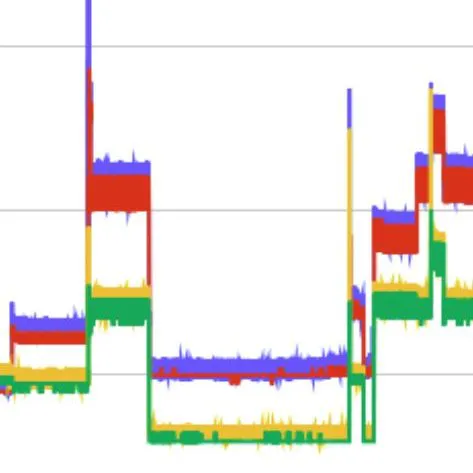Published

# Home Power Monitor

This device monitors the total current coming into your home and performs and FFT on it to determine what appliances are running.

IntermediateFull instructions provided4 hours3,240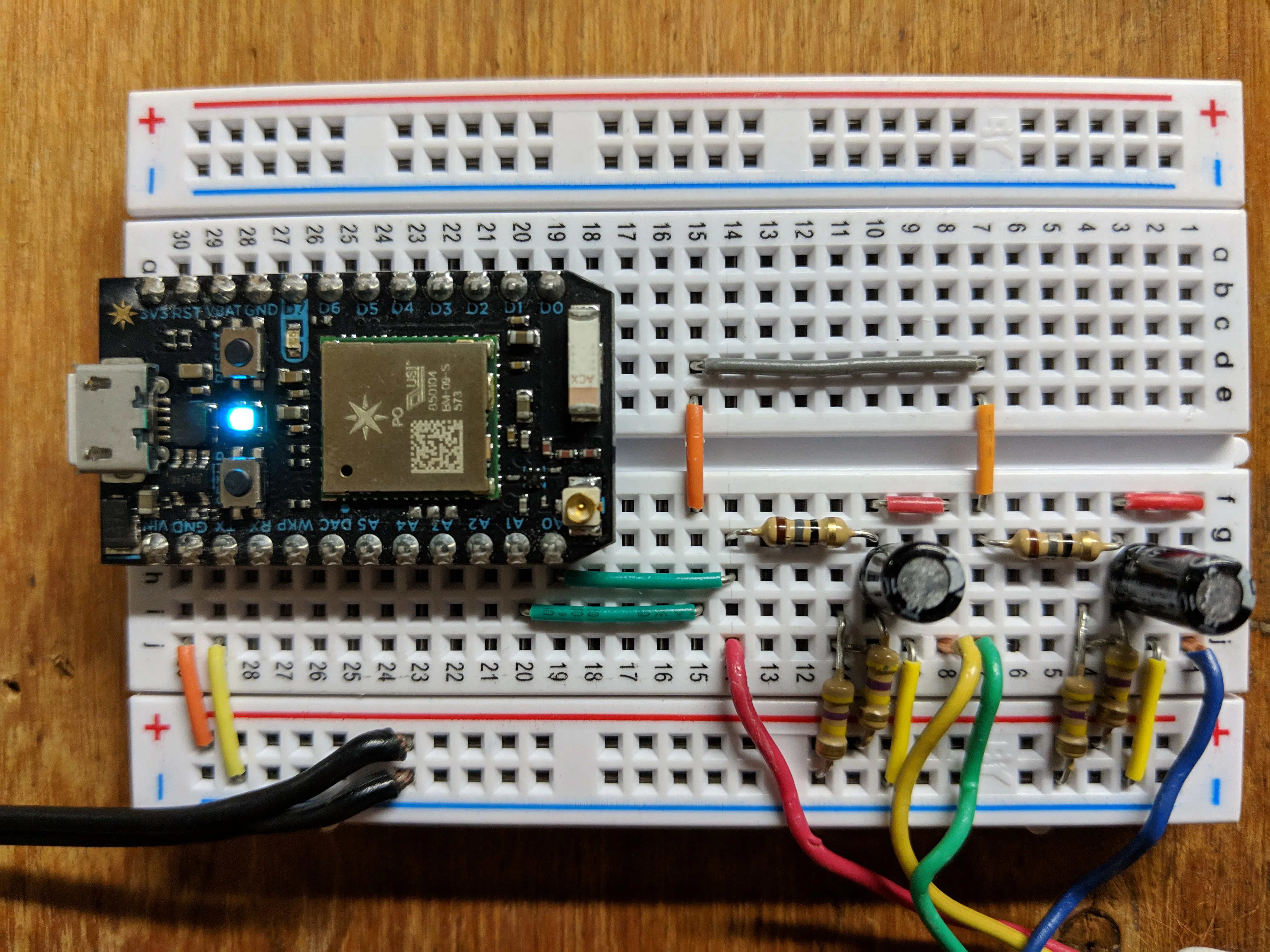## Things used in this project

### Hardware componentsParticle Photon
×1
 Current Transformer
×2
 Resistor 15 Ohm
×2
 Resistor 470 Ohm
×4×1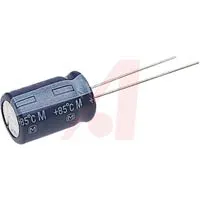Capacitor 10 µF
×2

### Software apps and online servicesParticle Build Web IDE## Schematics

### Voltage Divider Cicuit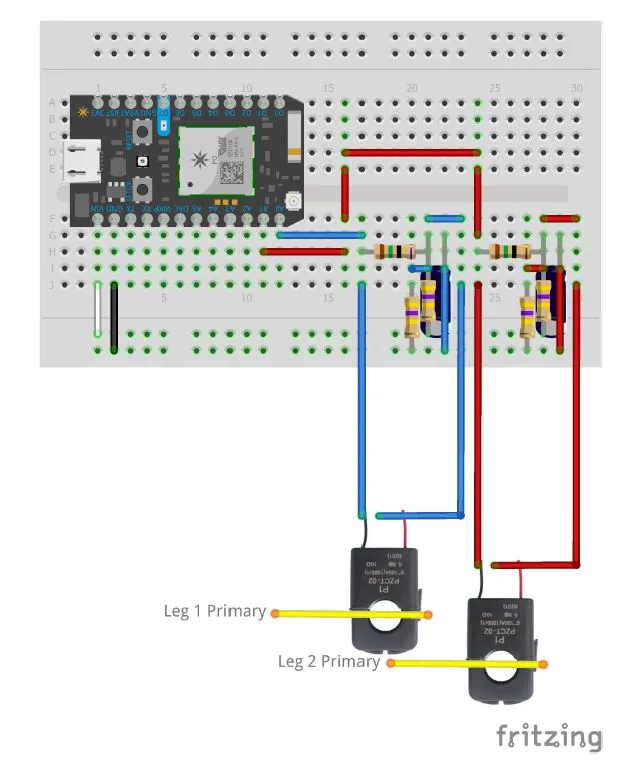### Photon Schematic

This is a schematic of how the photon can be wired. You have the option of creating only one voltage divider circuit if you like.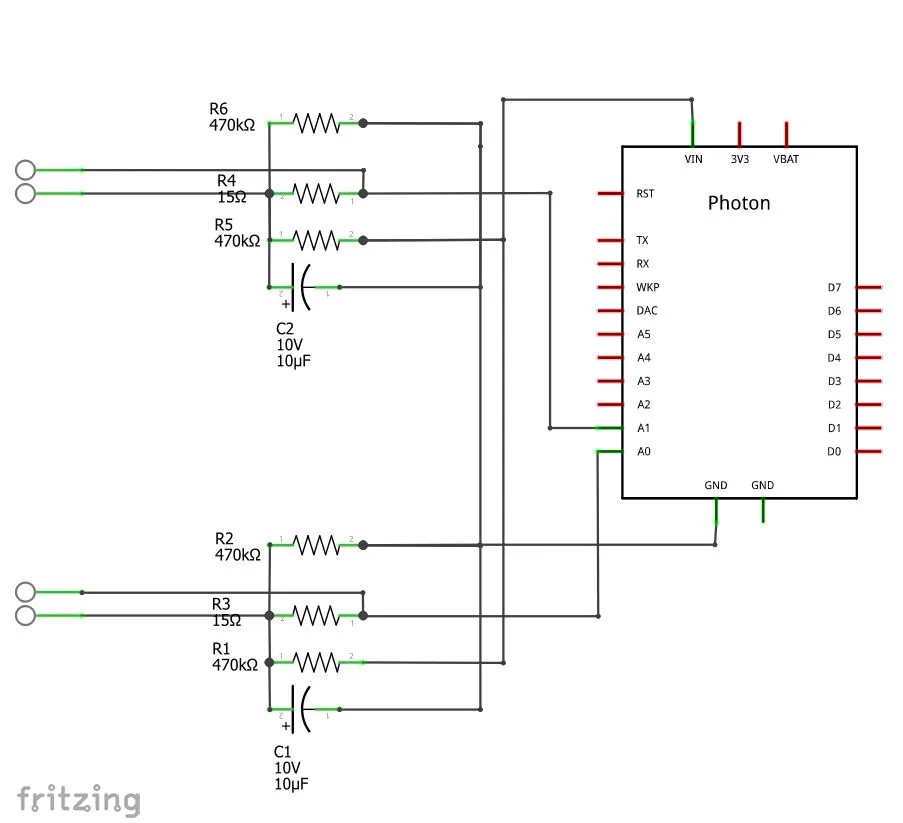## Code

JavaScript
This is the code to put into the Google Sheets Script that runs every night to calculate the total power used by each appliance.
```//  1. Enter sheet name where data is to be written below
var SHEET_NAME = "Sheet1";

//  2. Run > setup
//
//  3. Publish > Deploy as web app
//    - enter Project Version name and click 'Save New Version'
//    - set security level and enable service (most likely execute as 'me' and access 'anyone, even anonymously)
//
//  4. Copy the 'Current web app URL' and post this in your form/script action
//
//  5. Insert column names on your destination sheet matching the parameter names of the data you are passing in (exactly matching case)

var SCRIPT_PROP = PropertiesService.getScriptProperties(); // new property service

// If you don't want to expose either GET or POST methods you can comment out the appropriate function
function doGet(e){
return handleResponse(e);
}

function doPost(e){
return handleResponse(e);
}

function handleResponse(e) {
// shortly after my original solution Google announced the LockService
// this prevents concurrent access overwriting data
// we want a public lock, one that locks for all invocations
var lock = LockService.getPublicLock();
lock.waitLock(30000);  // wait 30 seconds before conceding defeat.

try {
// next set where we write the data - you could write to multiple/alternate destinations
var sheet = doc.getSheetByName(SHEET_NAME);

// we'll assume header is in row 1 but you can override with header_row in GET/POST data
var headers = sheet.getRange(1, 1, 1, sheet.getLastColumn()).getValues();
var nextRow = sheet.getLastRow()+1; // get next row
var row = [];
// loop through the header columns
if (headers[i] == "Timestamp"){ // special case if you include a 'Timestamp' column
row.push(new Date());
} else { // else use header name to get data
}
}
// more efficient to set values as [][] array than individually
sheet.getRange(nextRow, 1, 1, row.length).setValues([row]);
// return json success results
return ContentService
.createTextOutput(JSON.stringify({"result":"success", "row": nextRow}))
.setMimeType(ContentService.MimeType.JSON);
} catch(e){
// if error return this
return ContentService
.createTextOutput(JSON.stringify({"result":"error", "error": e}))
.setMimeType(ContentService.MimeType.JSON);
} finally { //release lock
lock.releaseLock();
}
}

function setup() {
SCRIPT_PROP.setProperty("key", doc.getId());
}

function Copy_delete() {
var date = Utilities.formatDate(new Date(), tz, 'MM-dd-yyyy');
sheets.setName(date);  // Rename second sheet
var rowString = "4:"+LastRow;

//deleting the data causes reference errors in the formulas in row 3, these lines restore them
};

function appliance_id () {
var last = spreadsheet.getSheets().length; //index of last sheet
var sheet = spreadsheet.getSheets(); //sheet with yesterday's data that we will be analyzing
var appliances = spreadsheet.getSheetByName("Appliances"); //sheet with appliance signatures
var LastApp = appliances.getLastRow(); //number of appliances
var sigRange = appliances.getRange('A2:W'+LastApp); //data range of appliance signatures
var sigs = sigRange.getValues(); //this creates 2 dimension array of appliance signatures
var LastRow = sheet.getLastRow(); //number of rows in yesterday's data
var dataRange = sheet.getRange('A4:W'+LastRow); //data range of yesterday's data
var data = dataRange.getValues(); //create 2 dimensional array of yesterday's data
var tolerance = .3; //how close harmonic deltas must be to signature to match appliance

for (i=0; i<LastApp-1; i++){ //loop for each appliance on appliance sheet, i will be row number on appliance sheet
sheet.insertColumnsAfter(sheet.getLastColumn(),1); //add new column to data sheet for this appliance
var col = sheet.getLastColumn()+1; //new column number
sheet.getRange(1,col).setValue(sigs[i]); //add the appliance name to new column
var flag = 0; //flag variable will be 0 for off and 1 for on
var totpower = 0; //this variable will accumulate total power used by appliance
for (j=2; j<=LastRow-4; j++){ //loop for each row of data, j will be row number on appliance sheet
var Acurrent_delta = data[j]-data[j-2]; //A phase current change
var Bcurrent_delta = data[j]-data[j-2]; //B phase current change
if (flag==0 && Math.abs(Acurrent_delta-sigs[i])<2 && Math.abs(Bcurrent_delta-sigs[i])<2) { //check current change and see if it's within 2 amps of this appliances signature
flag = 1; //if so, appliance may have turned on, so set flag to 1
for (k=1; k<23; k++){ //after matching current, then check harmonics, k will represent column numbers for both sheets
if (sigs[i][k]>1) { //only test the magnitude change if it's greater than 1, otherwise noise is too much
var magdelta = data[j][k]-data[j-2][k]; //magnitude change of harmonic being tested
if (Math.abs(sigs[i][k]-magdelta)>tolerance*sigs[i][k]) { //tests the magnitude change, if more than tolerance from signature sets to 0. Adjust tolerance to your taste
flag = 0;
}
}
}
}
var time = (data[j]-data[j-1])/3600000; //calculates the time in hours from last sample
var kwh = time*(sigs[i]+sigs[i])*120*flag/1000; //calculates the kWh of this sample
if (flag==1 && Math.abs(Acurrent_delta+sigs[i])<2 && Math.abs(Bcurrent_delta+sigs[i])<2) { //if flag is already 1, check for negative current change to see if appliance turned off
flag = 0;
}
sheet.getRange(j+4,col).setValue(kwh); //add kWh for this sample to appropriate cell
totpower+=kwh; //accumulate total kWh

}
sheet.getRange(3,col).setValue(totpower); //add total kWh consumed by appliance at top of appropriate column
}
}

function getDailyTotal() {
var LastRow = sheet.getLastRow()+1;
var date = yesterday_data.getName();
var Apow = yesterday_data.getRange('B3').getValue();
var Bpow = yesterday_data.getRange('M3').getValue();
var cost = .097; //this should be the cost/kWh for your utility company
var days2keep = 7; //this is how many days of data you wish to keep
sheet.getRange('A'+LastRow).setValue(date);
sheet.getRange('B'+LastRow).setValue(Apow);
sheet.getRange('C'+LastRow).setValue(Bpow);
sheet.getRange('D'+LastRow).setValue(Apow+Bpow);
sheet.getRange('E'+LastRow).setValue((Apow+Bpow)*cost);

//appliance totals
sheet.getRange('F'+LastRow).setValue(yesterday_data.getRange('X3').getValue());
sheet.getRange('G'+LastRow).setValue(yesterday_data.getRange('Y3').getValue());
sheet.getRange('H'+LastRow).setValue(yesterday_data.getRange('Z3').getValue());
//likewise add a row like this for each appliance

}
```

### Photon Program

C/C++
This is what the Photon runs in order to collect and process the current signature.
```// This #include statement was automatically added by the Particle IDE.
#include <EmonLib.h>

#include "math.h"

double IrmsA, IrmsB, lastIrmsA, lastIrmsB, diff;
const int A=0;  //analog channel for phase A
const int B=1;  //analog channel for phase B
const int samples=1024; //number of samples to collect for FFT
const int harm=10; //number of harmonics to store
const int sampling_freq=4096; //sampling frequency
const int base_harm=20; //lowest frequency to record
const int pause=5000; //amount of time in milliseconds to pause between each sample; recommend at least 5000
int res = sampling_freq/samples; //resolution (how many Hz are represented by each index)
int step = (base_harm/res); //used to grab harmonics in for loop

float current1[samples], current2[samples], imag1[samples], imag2[samples]; //arrays for FFT
float magA[harm], magB[harm];   //array of magnitudes from most recent FFT

unsigned int period;    //sampling period
unsigned long microseconds;

EnergyMonitor emon1;
EnergyMonitor emon2;

void setup() {
Serial.begin(115200);
period=round(1000000*(1.0/sampling_freq));  //calculate period based on frequency

Particle.variable("CurrentA", IrmsA); //exposes current data to Particle cloud
Particle.variable("CurrentB", IrmsB);

emon1.current(A,222); //the second parameter in this function is used to calibrate to your specific current transformer/burder resistor combo. You may want to use a clamp on meter to verify your actual current draw so your power usage is accurate, otherwise you may have inaccurate results.
emon2.current(B,97);//the second parameter in this function is used to calibrate to your specific current transformer/burden resistor combo. You may want to use a clamp on meter to verify your actual current draw so your power usage is accurate, otherwise you may have inaccurate results.
}

void loop() {
//collect samples for FFT

for (int i=0; i<samples; i++){
microseconds=micros();
imag1[i]=0;
imag2[i]=0;

while(micros() < (microseconds+period)){

}
}

//Run the FFT function (direction, power of 2, real array, imag array)

FFT(1,log2(samples),current1,imag1);
FFT(1,log2(samples),current2,imag2);

//compute magnitudes
for (int i=1;i<=harm;i++){
magA[i-1]=sqrt(current1[step*i]*current1[step*i]+imag1[step*i]*imag1[step*i]);
magB[i-1]=sqrt(current2[step*i]*current2[step*i]+imag2[step*i]*imag2[step*i]);
}

//calculate RMS current
IrmsA = emon1.calcIrms(2000);
IrmsB = emon2.calcIrms(2000);

//Convert magnitudes to strings to send to Google Sheets
String tIrmsA = String(IrmsA,2);
String A_1 = String(magA,2);
String A_2 = String(magA,2);
String A_3 = String(magA,2);
String A_4 = String(magA,2);
String A_5 = String(magA,2);
String A_6 = String(magA,2);
String A_7 = String(magA,2);
String A_8 = String(magA,2);
String A_9 = String(magA,2);
String A_10 = String(magA,2);

String tIrmsB = String(IrmsB,2);
String B_1 = String(magB,2);
String B_2 = String(magB,2);
String B_3 = String(magB,2);
String B_4 = String(magB,2);
String B_5 = String(magB,2);
String B_6 = String(magB,2);
String B_7 = String(magB,2);
String B_8 = String(magB,2);
String B_9 = String(magB,2);
String B_10 = String(magB,2);

//This event publishes data to Google Sheets

Particle.publish("GenericWebhookName","{\"IrmsA\":\"" + tIrmsA + "\",\"A1\":\"" + A_1 + "\",\"A2\":\"" + A_2 + "\",\"A3\":\"" + A_3 + "\",\"A4\":\"" + A_4 + "\",\"A5\":\"" + A_5 + "\",\"A6\":\"" + A_6 + "\",\"A7\":\"" + A_7 + "\",\"A8\":\"" + A_8 + "\",\"A9\":\"" + A_9 + "\",\"A10\":\"" + A_10 + "\",\"IrmsB\":\"" + tIrmsB + "\",\"B1\":\"" + B_1 + "\",\"B2\":\"" + B_2 + "\",\"B3\":\"" + B_3 + "\",\"B4\":\"" + B_4 + "\",\"B5\":\"" + B_5 + "\",\"B6\":\"" + B_6 + "\",\"B7\":\"" + B_7 + "\",\"B8\":\"" + B_8 + "\",\"B9\":\"" + B_9 + "\",\"B10\":\"" + B_10 + "\"}",5,PRIVATE);

delay(pause);

}

//FFT function from http://paulbourke.net/miscellaneous/dft/
short FFT(short int dir, int m, float *rx, float *iy) {

int n, i, i1, j, k, i2, l, l1, l2;
float c1, c2, tx, ty, t1, t2, u1, u2, z;

/* Calculate the number of points */
n = 1;
for (i=0;i<m;i++)
n *= 2;

/* Do the bit reversal */
i2 = n >> 1;
j = 0;
for (i=0;i<n-1;i++) {
if (i < j) {
tx = rx[i];
ty = iy[i];
rx[i] = rx[j];
iy[i] = iy[j];
rx[j] = tx;
iy[j] = ty;
}
k = i2;
while (k <= j) {
j -= k;
k >>= 1;
}
j += k;
}

/* Compute the FFT */
c1 = -1.0;
c2 = 0.0;
l2 = 1;
for (l=0;l<m;l++) {
l1 = l2;
l2 <<= 1;
u1 = 1.0;
u2 = 0.0;
for (j=0;j<l1;j++) {
for (i=j;i<n;i+=l2) {
i1 = i + l1;
t1 = u1 * rx[i1] - u2 * iy[i1];
t2 = u1 * iy[i1] + u2 * rx[i1];
rx[i1] = rx[i] - t1;
iy[i1] = iy[i] - t2;
rx[i] += t1;
iy[i] += t2;
}
z =  u1 * c1 - u2 * c2;
u2 = u1 * c2 + u2 * c1;
u1 = z;
}
c2 = sqrt((1.0 - c1) / 2.0);
if (dir == 1)
c2 = -c2;
c1 = sqrt((1.0 + c1) / 2.0);
}

/* Scaling for forward transform */
if (dir == 1) {
for (i=0;i<n;i++) {
rx[i] /= n;
iy[i] /= n;
}
}

return(0);
}
```

## Credits

### Terabull

1 project • 0 followers

### Keith Snyder

0 projects • 0 followers